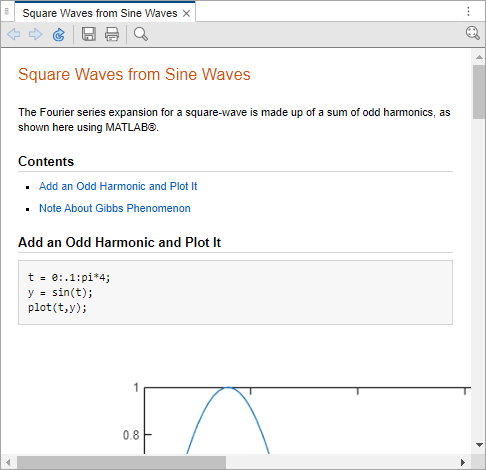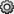## Web 浏览器和 MATLAB

### 关于 Web 浏览器和 MATLAB

• MATLAB Web 浏览器

• 帮助浏览器

• 您的系统 Web 浏览器，例如 Mozilla® Firefox®

MATLAB 使用不同的浏览器显示不同类型的信息：

• 网站显示在系统浏览器中。

• 文档显示在帮助浏览器中。

• 其他 HTML 文件显示在 MATLAB Web 浏览器中。例如，在将 MATLAB 程序文件发布到 HTML 后，HTML 文件会显示在 MATLAB Web 浏览器中：#### MATLAB Web 和帮助浏览器

MATLAB Web 浏览器和帮助浏览器可能不支持特定网站或 HTML 页面使用的所有功能。例如，MATLAB Web 浏览器不显示 `.bmp`（位图）图像文件。对 HTML 页面中的图像文件，请改用 `.gif``.jpeg` 格式。

#### 系统浏览器

MATLAB 使用的系统浏览器取决于您的平台：

• 在 Microsoft® Windows®Apple Macintosh 平台上，MATLAB 使用操作系统的默认浏览器。

• 在 UNIX® 平台上，MATLAB 使用 Mozilla Firefox 浏览器。可以使用 Web 预设项为 MATLAB 指定其他系统浏览器。

### 指定用于连接到 Internet 的代理服务器设置

• MATLAB 支持非身份验证、基本、摘要式和 NTLM 代理身份验证类型。

• 如果指定具有基本身份验证的代理，则 MATLAB 仅支持 HTTP 连接，不支持 HTTPS 连接。

• 不能使用脚本指定代理服务器设置。

• 没有自动方法可向 MATLAB 提供您的系统浏览器使用的代理服务器设置。

1. 主页选项卡的环境部分，点击。选择 MATLAB > Web

2. 选中使用代理服务器连接到 Internet 复选框。

3. 代理主机代理端口指定值。

下面是主机的可接受格式的示例：`172.16.10.8``ourproxy`。对于端口，仅输入整数，例如 `22`。如果您不知道代理服务器的这些值，请向您的系统管理员或网络管理员询问相关信息。

如果您的代理服务器需要用户名和密码，请选中使用包含身份验证的代理复选框。然后输入您的代理服务器的用户名和密码。

4. 通过点击按钮来确保您的设置工作正常。

MATLAB 尝试连接到 `https://www.mathworks.com`

• 如果 MATLAB 可以访问 Internet，则会在此按钮旁边显示成功!

• 如果 MATLAB 无法访问 Internet，则会在此按钮旁边显示失败!。更正所输入的值并重试。如果仍然无法连接，请尝试使用在对 MATLAB 许可证进行身份验证时使用的值。

5. 点击以接受更改。

6. 重新启动 MATLAB 以启用更改。

### 为 Linux 平台指定系统浏览器

1. 主页选项卡的环境部分，点击。选择 MATLAB > Web

2. 系统 Web 浏览器下的命令字段中，指定用于打开浏览器的系统命令，例如 `opera` 可打开 Opera Web 浏览器。

3. 选项字段中添加用于打开系统浏览器的选项。例如，`geometry 1064x860` 指定 Opera 的窗口大小。

4. 点击